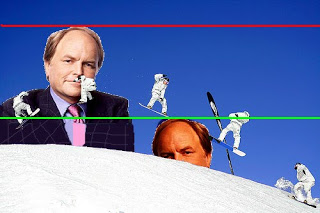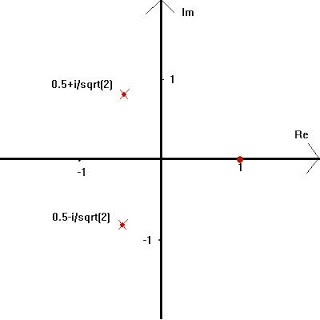--- title: Divergence and reduction with complex numbers --- I came across this introduction to the concept of "imaginary" numbers, and thought it would be worthwhile to write a post about how cool complex and imaginary numbers actually are. If you don't know what an imaginary number or a complex number is then don't worry. That link provides quite a nice intro, and I'll give my own here: An "imaginary" number is a number which doesn't fit anywhere on the number line. No matter how closely you zoom in, or how far along you go, you'll never find it. That's why they're called "imaginary", even though it is an unfortunate name as the other blog points out. Before you dismiss imaginary numbers as useless, let's consider another set of numbers which is made up and doesn't exist in the real world: negative numbers. Negative numbers cannot exist like the natural (positive) numbers do. You cannot have -5 apples. Nevertheless, negative numbers are useful for made up mathematical worlds, like banking for example, which can have a definite impact on the real, physical world. Negative numbers also arise in situations involving symmetry, relative values and arbitraryness. Let's take a realistic example: if we think of positions, there is no set of coordinate axes in the physical world, and the whole Universe is symmetric spatially, so we can just make up the numbers we use to be anything we find convenient. Let's say we've got a chocolate bar:We'd like to be able to refer to each section of chocolate in a unique way, so we can say that the bottom left section is at position (0,0), and define our unit of length to be one chocolate section. Thus we can say "The sections at (0,2) and (0,3) have been bitten". Now let's say that Dr Ed Daw has had a busy day and didn't have chance to grab some food. He's so hungry that he doesn't have enough energy to move more than one unit of distance to get the food he needs, but he spies our chocolate bar. Can Dr Daw get the food he needs?:To find the distance between the doctor and the chocolate we need to know the doctor's position. We can't use a positive number to define the position, since even though there are an infinite number of them, they get less and less accurate the further we look. Instead we have to use a negative number, allowing us to say that Dr Ed Daw is at position (0, -1). We know there is chocolate at (0,0), so to get from his position to the food only takes one unit of distance, since (0,-1)+(0,1)=(0,0). The only reason we had to use a negative number here is because of the way we defined our mathematical model of the situation. There's no such thing as "negative position", but when we used the doctor's negative position to find the distance he has to move, which is a physical quantity, the negative numbers disappeared and we got a meaningful, and correct, answer. So let's move on to imaginary numbers: Close to the surface of the Earth, gravity is pretty constant, with a value of 9.81 metres per second per second. This means that vertical speed (metres per second, miles per hour, etc.) will go down by 9.81 metres per second (if it's pointing up), or up by 9.81 metres per second (if it's pointing down) every second. Speed describes how your position changes in time (eg. how many metres you move in a second), so the position of something flying through the air will change by the speed which itself will change due to gravity, so the position will curve. The shapes made by these curves are called parabolas and behave like the quantity -Ax^2 + Bx + C, where x is your horizontal position (or you could use time here instead) and A, B and C are numbers specific to what you're looking at. We get a minus sign for the x^2 because gravity points down and we define up to be positive. If we want to know at what position or time a ballistic (falling) object will reach a certain height, what we're really asking is at what position or time does Ax^2 + Bx + C equal the height we're interested in. We can do this with an example: Dr Daw has finished his chocolate and is trying to show off some lasers to his younger, more tanned brother. They each attach a laser to their head, Ed takes the red one and his brother De takes the green one. The lasers need to be kept cool, so they go to the Sheffield Ski Village. While they watch the skiers go past, De asks his brother "When do the skiers get higher than the lasers attached to our heads?". How can Ed work this out, given that the skiers motion can be described by -x^2 + 0x + 5, that De's green laser is a height of 4 and that Ed's red laser is at a height of 6?Ed knows that he can take the skiers's motion and, using the apex of the jump as position 0, work our at which positions they get above the lasers by saying -x^2 + 5 = 4 when they get above De's laser and -x^2 + 5 = 6 when they cross his laser. Working out the first gives the valid positions, x, as: -x^2 + 5 = 4
-x^2 = 4 - 5
-x^2 = -1
x^2 = 1
x = +1 or -1 He tells his brother that the skiers will cross the green laser at positions -1 and +1, since they satisfy the equation of motion. He then tries to work out when they cross the red laser: -x^2 + 5 = 6
-x^2 = 6 - 5
-x^2 = 1
x^2 = -1 Then Ed gets stuck, since there's no positive or negative number he can think of which would fit as x here. Thus he declares to De "There are no solutions, so the skiers never get above my laser.". De is not satisfied with this. He says "That's cheating. I want you to finish working it out.". Ed knows how stubborn his brother is, since he carried on wearing fake tan even after going on "Snog, Marry, Avoid" the previous year, so he invents a number to satisft this equation: "The skiers cross my laser whenever they get to a position which I will call N. They will also cross the laser if they get to a position -N. Thus, +N and -N are the solutions to this equation." De is still not satisfied, so he asks his brother what N is. The doctor then explains imaginary numbers to the younger man: "N is a quantity that is not a positive number, or a negative number, however it is a very useful quantity, since it lets us solve the equation for my laser. You can't find it no matter how closely you look between the numbers, or how far along you go. This means that its value is orthogonal to the real numbers, since the two are independent, in the same way that you can't change an x coordinate by moving in the y direction. In fact, we could draw a diagram using the real numbers as an x axis and these imaginary ones as the y axis." "I'm confused." said De, "Will you please draw me such a diagram?" "I can't be arsed," Ed replied, "so here's one I found on Wikipedia."Using this diagram, Ed could explain to his brother how a Real number (Re) sits on the horizontal line, how an Imaginary number (Im) sits on the vertical line, and how the quadrants are filled with numbers that have an imaginary part and a real part, and are called Complex numbers. De seemed to understand, but had some questions: "OK, I'll accept what you just said, but then where does our quantity N fit in this diagram? Also, there are some undefined variables; what is the value of the "i" that's written on the diagram?" "The "i" represents the Imaginary unit." Ed explained, "It's equivalent to the number 1 for natural numbers, and -1 for negative numbers. Every Imaginary number is made out of some quantity of i." "So what is the value of i?" "The imaginary unit is defined by the equation i^2 = -1. No more, no less." "But that's the equation for the skiers crossing your laser! That means that N = i and the skiers will cross if they ever get to a position i, which they can never do because imaginary space is orthogonal to physical space, so no force can ever push them off the Real lines for x, y and z." "Well done" said an impressed Ed "but you're forgetting how to do square roots! There are always two square roots of a number, just like we had +N and -N. That means that i^2 = -1 and (-i)^2 = -1. So tell me, does N = i or does N = -i?" De thought about this for a minute before declaring "There's no way of telling! If N = i then N^2 = -1 since i^2 = -1, but also (-N)^2 = -1 since the minus signs cancel so -N = i. But also, (-i)^2 = -1 so N = -i and, because the minus signs cancel, i^2 = -1, so N = i. So N = +i or -i and -N = +i or -i, they're equal!" "Don't be too hasty to call them equal!" cautioned Ed "If N = -N then i = -i and I could do the following: i = -i This is what you are claiming
i + i = -i + i We can add i to each side to preserve the equality
2i = 0 Adding i to i gives 2i, whilst adding i to -i gives zero
i = 0 We can divide both sides by 2 and preserve the equality
i*i = 0*i We can multiply each side by i and preserve the equality
i*i = 0*0 We showed that i=0 so use that on the right hand side
i*i = 0 We know 0*0=0